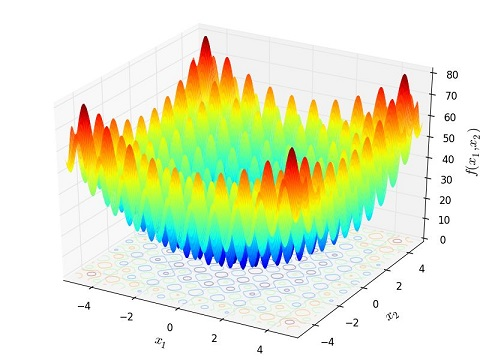# 为什么要采样？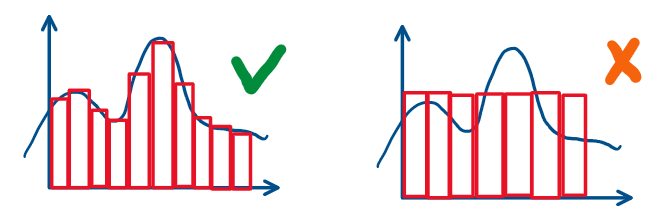# 怎么采样？

## 概率分布采样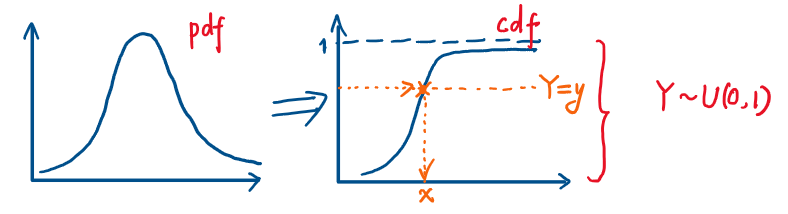## 重要性采样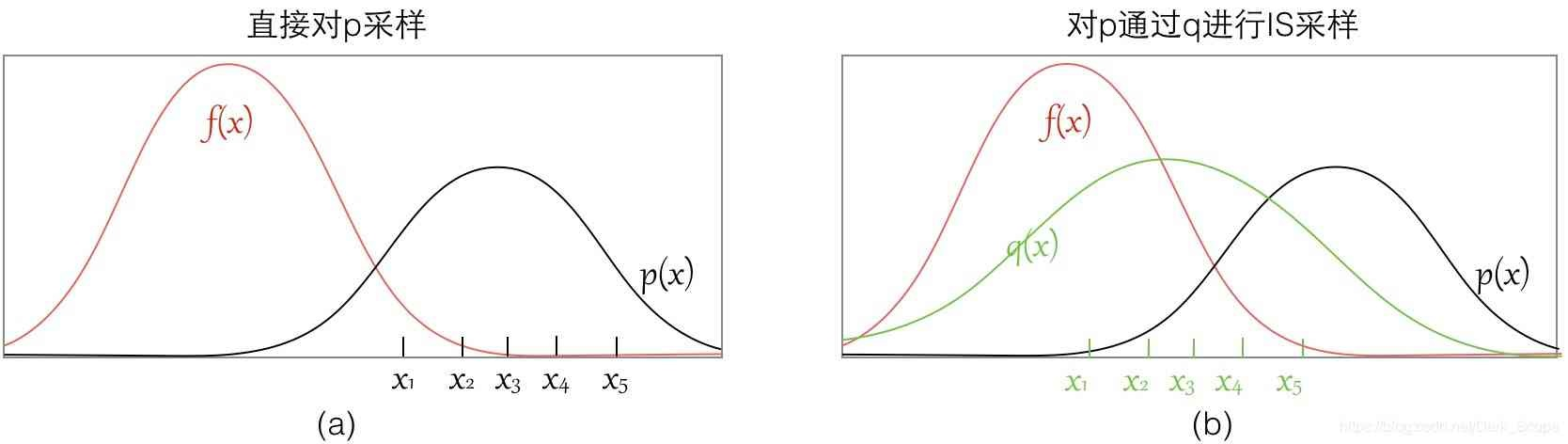## MCMC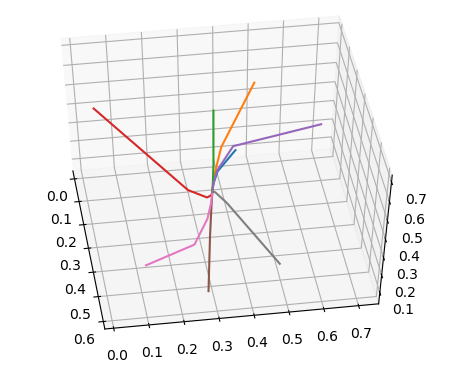### M-H（Metropolis-Hastings）

M-H是MCMC的一种实现。回顾一下，上面这个细致平衡的等式是怎么的来的呢？第一步，也是最关键的是找到了一个满足马尔可夫性质的转移矩阵M，于是才有了之后的平稳分布，也就最终能达到细致平衡条件。

M-H的做法十分大胆，它拿一个和目标转移矩阵一样大的随机马尔可夫转移矩阵Q作为转移矩阵的一部分。显然此时$\pi(x)Q(y|x) \neq \pi(y)Q(x|y)$。而后，为了让等式相等，引入类似拒绝采样里提到的接受率这样一个概念。令$\alpha(x,y) = min⁡(1,\frac{\pi(x)Q(y|x)}{\pi(y)Q(x|y)})$，于是一个细致平衡就被构造出来了

1. 输入需要采样的平稳分布$\pi$，转移的次数n；
2. 随机构造一个马尔可夫转移矩阵Q；
3. 挑选一个初始状态$x_0$；
4. for t = 1 to n：
1. 采样$x^∗ \sim Q(x|x_{t−1})$；
2. 从均匀分布中采样$u \sim U(0,1)$；
3. if u < $\alpha(x_{t−1},x^∗)$：接受转移，令$x_t = x^∗$；
4. else: 不转移，令$x_t = x_{t−1}$；
5. 返回采集到的一系列x；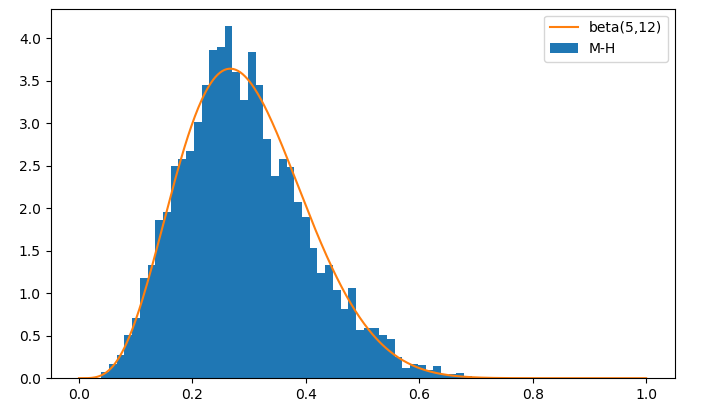### Gibbs

Gibbs适用于更高维的分布的采样，并且用一句话概括它的思想就是：固定其他维度上的随机变量，那么剩下那一维上的随机变量仍然满足细致平衡。

1. 给定一个平稳分布$\pi(x_1,x_2)$，给定转移次数n；
2. 随机初始化一个点$X_0(x_1^0,x_2^0)$；
3. for t = 1 to n：
1. 固定$x_1$，采样$x_2$，得到$x_2^{t+1} \sim \pi(x_2|x_1^t)$；
2. 固定$x_2$，采样$x_1$，得到$x_1^{t+1} \sim \pi(x_1|x_2^t)$；
3. 得到新采样点$X_{t+1}(x_1^{t+1}, x_2^{t+1})$；
4. 返回采集到的一系列X；